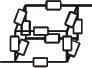# Total Resistance in this Circuit

• AROD

## Homework Statement

I have a network of resistors in a box-formation. There is 1 resistor of equal resistance in between every corner of the box, so 12 in total.

## Homework Equations

In series R = r1 + r2 + ... ri
In parallel, 1/R = 1/r1 + 1/r2 + ... 1/ri

## The Attempt at a Solution

I can't really draw a 2D analogous network due to the connections. I know kirchhof's rules must be applied somehow... that no current will flow between two points of equal potential difference, but not sure where that would be.

#### Attachments

•KomplexSchaltung.jpg
2.2 KB · Views: 422
Just apply kirchhof law, that the sum of currents at node is zero. So first introduce some current in the circuit by applying a voltage source of 1 volt to make it easy. Then analyze every node to see how the currents are distributed in the branches.

first node brances to 3, each of those branch twice, 6 branches then converging on 3 nodes, which tnen go back to 1 node.

since all resistors are equal, can the 3 node to 3 node connection be just expressed as 3 parallel with 2 each? my reaction is to make each little group of 2 parallel, giving a total of 5/6 for the entire thing, but i don't know.

this is also then accounting for all of the resistors contributing. it looks to me like charge would flow through everything.